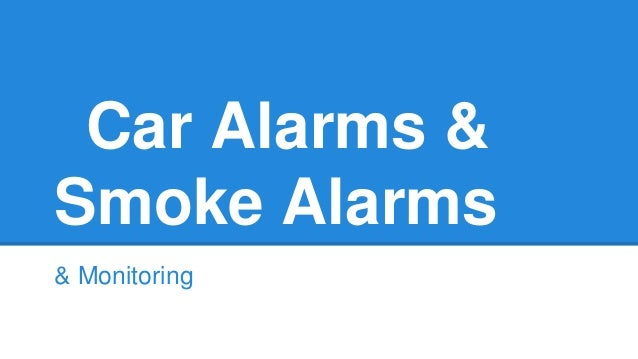Successfully reported this slideshow.Upcoming SlideShare
×

# Car Alarms & Smoke Alarms [Monitorama]

6,873 views

Published on

Nobody likes false negatives. When your Nagios probes fail to detect a problem, it can hurt your sales, your reputation, and even your ego (especially your ego). The solution: tune the thresholds. Right? You can handle a couple spurious late-night pages if it means you’ll reliably detect real failures.

I will argue that – while easy – exchanging false negatives for false positives does more harm than good. Borrowing the medical concepts of specificity and sensitivity, I’ll show how deceptive this tradeoff can be. I’ll also make the case that putting in the extra effort to minimize both types of falsehoods is necessary and healthy. When the alarm goes off, you shouldn’t have to spend precious minutes sniffing for smoke.

Published in: Technology, Education
• Full Name
Comment goes here.

Are you sure you want to Yes No• Be the first to comment

### Car Alarms & Smoke Alarms [Monitorama]

1. 1. Car Alarms & Smoke Alarms & Monitoring
2. 2. Who’s this punk? • Dan Slimmon • @danslimmon on the Twitters • Senior Platform Engineer at Exosite • Previously Operations Team Manager at Blue State Digital
3. 3. Learn to do some stats and visualization. You’ll be right much more often, & people will THINK you’re right even more often than that!
4. 4. Signal-To-Noise Ratio
5. 5. A word problem You’ve invented an automated test for plagiarism.
6. 6. • Plagiarism: 90% chance of positive • No Plagiarism: 20% chance of positive • Jerkwad kids plagiarize 30% of the time A word problem
7. 7. Question 1 Given a random paper, what’s the probability that you’ll get a negative result? • Plagiarism: 90% chance of positive • No Plagiarism: 20% chance of positive • 30% chance of plagiarism
8. 8. Question 2 If there’s plagiarism, what’s the probability PLAJR will detect it? • Plagiarism: 90% chance of positive • No plagiarism: 20% chance of positive • 30% chance of plagiarism
9. 9. Question 2 If there’s plagiarism, what’s the probability you’ll detect it? • Plagiarism: 90% chance of positive • No plagiarism: 20% chance of positive • 30% chance of plagiarism
10. 10. Question 3 If you get a positive result, what’s the probability that the paper is plagiarized? • Plagiarism: 90% chance of positive • No plagiarism: 20% chance of positive • 30% chance of plagiarism
11. 11. No Plagiarism Plagiarism
12. 12. No Plagiarism Negative Positive
13. 13. No Plagiarism Negative Positive Plagiarism Negative Positive
14. 14. Question 1 Given a random paper, what’s the probability that you’ll get a negative result?
15. 15. No Plagiarism Negative Positive Plagiarism Negative Positive
16. 16. Question 2 If the paper is plagiarized, what’s the probability that you’ll get a positive result?
17. 17. No Plagiarism Negative Positive Plagiarism Negative Positive
18. 18. Question 3 If you get a positive result, what’s the probability that the paper was plagiarized?
19. 19. No Plagiarism Negative Positive Plagiarism Negative Positive
20. 20. Question 3 If you get a positive result, what’s the probability that the paper was plagiarized? Dark Green ------------------------------------------ (Dark Blue) + (Dark Green)
21. 21. Question 3 If you get a positive result, what’s the probability that the paper was plagiarized? 27 ------------------------------------------ 14 + 27
22. 22. Question 3 If you get a positive result, what’s the probability that the paper was plagiarized? 65.8%
23. 23. Sensitivity & Specificity Sensitivity: % of actual positives that are identified as such Specificity: % of actual negatives that are identified as such
24. 24. Sensitivity & Specificity Sensitivity: High sensitivity Test is very sensitive to problems Specificity: High specificity Test works for a specific type of problem
25. 25. Specificity: Probability that, if a paper isn’t plagiarized, you’ll get a negative. Sensitivity & Specificity Sensitivity: Probability that, if a paper is plagiarized, you’ll get a positive. 90% 80%
26. 26. Specificity Sensitivity Prevalence
27. 27. http://i.imgur.com/ LkxcxLt.png
28. 28. Positive Predictive Value The probability that If you get a positive result, Then it’s a true positive.
29. 29. When you get paged at 3 AM, Positive Predictive Value is the probability that something is actually wrong.
30. 30. Imagine if you will... • Service has 99.9% uptime • Probe has 99% sensitivity • Probe has 99% specificity
31. 31. Pretty decent, right?
32. 32. Let’s calculate the PPV.
33. 33. True Negative False Negative False Positive True Positive Positive Result Negative Result Condition Present Condition Absent
34. 34. The true-positive probability P(TP) = (prob. of service failure) * (sensitivity) P(TP) = 0.1% * 99% P(TP) = 0.099% Let’s calculate the probability that any given probe run will produce a true positive.
35. 35. The true-positive probability P(TP) = 0.099% So roughly 1 in every 1000 checks will be a true positive.
36. 36. The false-positive probability P(FP) = (prob. working) * (100% - specificity) P(FP) = 99.9% * 1% P(FP) = 0.99% So roughly 1 in every 100 checks will be a false positive.
37. 37. Positive predictive value PPV = P(TP) / [P(TP) + P(FP)] PPV = 0.099% / (0.099% + 0.99%) PPV = 9.1% If you get a positive, there’s only a 1 in 10 chance that something’s actually wrong.
38. 38. Why is this terrible?
41. 41. You want smoke alarms, not car alarms.
44. 44. Why do we have such noisy checks?
45. 45. “Office Space”, 1999.
46. 46. Monty Python’s Flying Circus, 1975.
47. 47. Semi-Practical Advice Undetected outages are embarrassing, so we tend to focus on sensitivity. That’s good. But be careful with thresholds.
48. 48. Semi-Practical Advice Response Time Threshold Positive Predictive Value
49. 49. Semi-Practical Advice Get more degrees of freedom.
50. 50. Semi-Practical Advice Response Time Threshold Positive Predictive Value
51. 51. Semi-Practical Advice Hysteresis is a great way to add degrees of freedom. • State machines • Time-series analysis